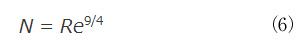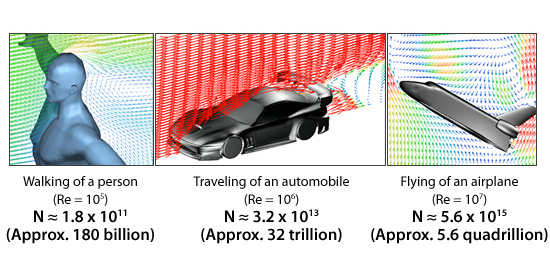# Basic Course of Thermo-Fluid Analysis 19: Chapter 5 Basics of thermo-fluid analyses - 5.9.3 Direct Numerical Simulation (DNS)

#### 5.9.3 Direct Numerical Simulation (DNS)

Directly solving the governing equations of motion without modeling (which is an approximation) is the simplest and most exact method for analyzing a turbulent flow. This method is called Direct Numerical Simulation (DNS).
The principle of DNS is simple; however, an extremely fine mesh is required to solve the flow exactly. The mesh must be fine enough to resolve the smallest scale eddies that were generated from larger eddies reiteratively splitting. The smallest scale of eddies is called the Kolmogorov scale and is determined by the degree of fluid viscosity. A flow with a large Reynolds number is less affected by the viscosity, and the scale of smallest eddies will be smaller. Therefore, the number of elements in the mesh will be greater. The number of elements required for DNS in 3D can be estimated by the following equation:where,

N: Number of elements
Re: Reynolds number

We can apply equation (6) to three types of flow. Figure 5.26 shows the calculation results for each flow.Figure 5.26: Relation between Reynolds number and the number of elements

The results show that billions of elements will be required to solve for the flow using DNS even when the Reynolds number of a flow is relatively small.
With current computer software and hardware technology, DNS cannot yet be practically implemented. Therefore, DNS is mainly used today for research-purposes, while RANS and LES, which were discussed previously, are used for modeling in practical simulations.

#### Conclusion

Over the past 30 years, CFD has gradually become a tool that is crucial for product design and manufacturing simulation. Substantial improvements in calculation methods and computer hardware performance have made practical use of CFD as a design tool a reality. As a result, more and more engineers include CFD software as a part of their standard toolset. CFD is a complicated subject and mastering it is not easy. However, we hope this course helps take some of the mystery out of CFD and better prepares you as you enter this exciting field.